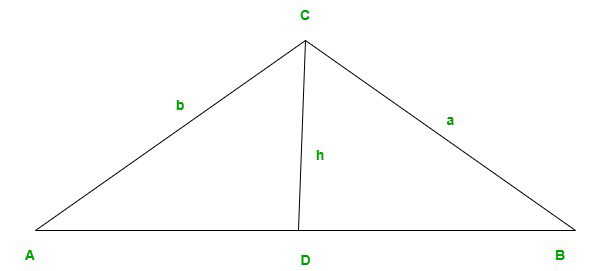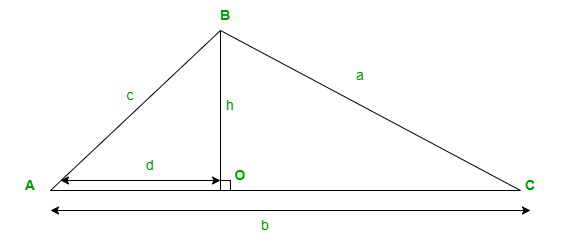Open in App
Not now

# Laws of Sines and Cosines Formula

• Last Updated : 18 Feb, 2022

Trigonometry is a branch of mathematics that is concerned with the concept of evaluation of angles. Mostly, these angles are measured in a right-angled triangle. The trigonometric functions are derived through trigonometric operations which are carried out with the sides and angles of a right angle triangle respectively.

The given article is concerned with a sub-topic of trigonometry. The article explains the law of the sines formula and the law of cosine formula, and the content also includes an explanation about trigonometric ratios and their trigonometric values with respect to various angles.

### What are Trigonometric Ratios?

Trigonometric ratios are the values of trigonometric functions given in numerical forms and are derived in ratios with respect to the sides and angles of the given right-angled triangle. There are three sides in an aright angled triangle viz. hypotenuse, perpendicular, and base on which the ratios depend upon. The six basic functions of trigonometry which give different numerical values under different angles are sine, cosine, tangent, cosecant,  secant, and cotangent.

The trigonometric values of these functions under different angles are given below:

### Law of Sines Formula

The Law of Sines Formula or Sine rule is understood as the ratio of sides and equivalent angles of a given triangle. The law of sines formula is generally used to evaluate the unknown sides or angles off an oblique triangle.

The mathematical formula of the law of sines is given aswhere, a, b and c are the sides of the triangle.

In a trigonometric operation where the sine rule is used, we generally consider at least two angles of the triangle.

Derivation of the law of sines formula

A right-angled triangle is considered to prove the formula of the sine rule.

So, let us suppose a triangle ABC with its respective sides

AB = c

BC = a

and, AC = bThen, draw a perpendicular CD from the base of the triangle i.e. AB. Hence, the height of the given triangle will be CD = h.

Now, the drawn perpendicular will divide the triangle into two right-angled triangles that will be CDB and CDA.

Here, to evaluate a/b=sinA/SinB

In CDA

=>sinA=h/b

And, In CDB

=>sinB=h/a

Then,

=>sinA/sinB=(h/b)/(h/a)

Therefore,

=>SinA/sinB=a/b proved.

Similarly, the other functions as sinB/sinC = b/c can also be proved with the application of the same method.

Some of the other formulas of The sine rule

• a:b:c = sinA:sinB:sinC
• a/b = sinA/sinB
• b/c = sinB/sinC

### Law of Cosine Formula

The law of cosine or cosine rule is the expression that relates the lengths of sides and cosines of angles of the given triangle. The law of cosine states that “the square of any one side of a triangle is equal to the difference between the sum of squares of the other sides and double the product of other sides and cosine angle included between them.”

Mathematically, the law of cosine is expressed as

a2 = b2+ c2 – 2bc. cosA

b2 = c2 + a2 – 2ca. cosB

c2 = a2 + b2 – 2ab. cosC

where,

a,b, and c are the lengths of the sides

And, A, B, and C are the angles.

Derivation of Cosine Law

Let us suppose a triangle ABC with its respective sides

AB = c

BC = a

and, AC = bThen, draw a perpendicular OB from the base of the triangle i.e. AC. Hence, the height of the given triangle will be OB=h.

Now, the drawn perpendicular will divide the triangle into two right-angled triangles that will be ABO and BOC.

Now, In ABO

sinA=BO/AB=h/c…………..(i)

and,

cosA=AO/AB=d/c ………….(ii)

From equations (i) and (ii) we get the value of h and d,

h=c(sinA)

d=c(cosA)

By using the Pythagoras theorem in BOC

=>a2=h2+(b-d)2

Substituting the values of h and d,we get

=>a2=c2sin2A+b2+c2cos2A-2bc. cosA

=>a2=c2(sin2A+cos2A)+b2-2bc.cosA

=>a2=c2+b2-2bc. cosA proved.

Similarly the other expressions

b=c+a-2ca.cosB and

c=a+b-2ab.cosC can also be proved by the same method.

### Sample Problems

Problem 1: Find the missing side of a triangle having its two sides 12cm and 8cm with the angle between them measuring 60 degrees.

Solution:

Given

Let the two sides b and c be 12cm and 8cm respectively and a be the missing side.

The angle between b and c is 60°

Now, by using the law of cosine formula

a2=b2+c2-2bc. cosA

=>a2=(12)2+(8)2-2(12)(8)cos60°

=>a2=144+64-192×0.5

=>a2=208-96

=>a2=112

=>a=10.58cm

Problem 2: Find the missing side of a triangle having its two sides 25cm and 10cm with the angle between them measuring 30degrees.

Solution:

Given

Let the two sides b and c be 25cm and 10cm respectively and a be the missing side.

The angle between b and c is 30°.

Now, by using the law of cosine formula

a2=b2+c2-2bc. cosA

=>a2=(25)2+(10)2-2(25)(10)cos30°

=>a2=625+100-500×0.86

=>a2=725-430

=>a2=295

=>a=17.17cm

Problem 3: Find the missing side of a triangle having its two sides 21cm and 14cm with the angle between them measuring 45degrees.

Solution:

Given

Let the two sides b and c be 21cm and 14cm respectively and a be the missing side.

The angle between b and c is 45°.

Now, by using the law of cosine formula

a2=b2+c2-2bc. cosA

=>a2=(21)2+(14)2-2(21)(14)cos45°

=>a2=441+196-588×.707

=>a2=637-415.7

=>a2=221.3

=>a=14.87cm

Problem 4: Find the value of side a, if the two angles are ∠A=65° and ∠B=40° and b=12cm.

Solution:

Given

∠A=65° and ∠B=40°

And, b=12cm

Now,

a/sinA=b/sinB

=>a/sin65°=12/sin40°

=>a/0.906=12/0.642

=>a=16.93cm.

Problem 5: Find the value of side a, if the two angles are ∠A=72° and ∠B=60° and b=6cm.

Solution:

Given

∠A=72° and ∠B=60°

And, b=6cm

Now,

a/sinA=b/sinB

=>a/sin72°=6/sin60°

=>a/0.951=6/0.86

=>a=6.41cm.

Problem 6: Find the value of side a, if the two angles are ∠A=48° and ∠B=35° and b=16cm.

Solution:

Given

∠A=48° and ∠B=35°

And, b=16cm

Now,

a/sinA=b/sinB

=>a/sin48°=16/sin35°

=>a/0.743=16/0.57

=>a=20.85cm.

My Personal Notes arrow_drop_up